## The Fast Fourier Transform

The Fast Fourier Transform computes the DFT quickly. This important algorithm for computer science (not only computer algebra, but also digital signal processing for instance) was (re)-discovered in 1965 by Cooley and Tukey.

Let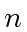be a positive even integer,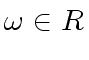be a primitive-th root of unity and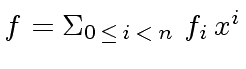. In order to evaluate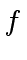at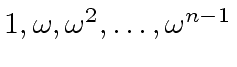, we follow a divide-and-conquer strategy; more precisely, we consider the divisions with remainder ofby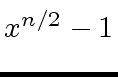and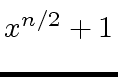. So let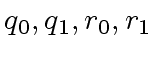be polynomials such that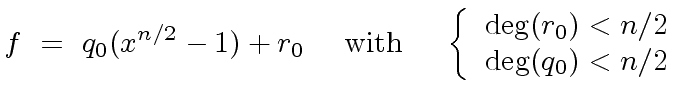(31)

and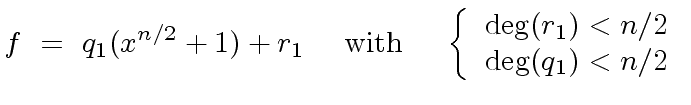(32)

The relations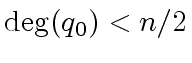and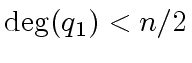hold because the polynomialhas degree less than. Observe that the computation of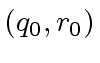and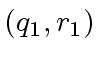can be done very easily. Indeed, let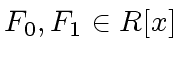be such that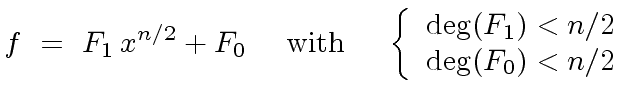(33)

We have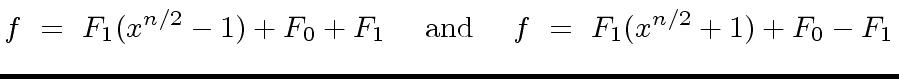(34)

Hence we obtain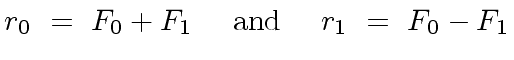(35)

Let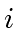be an integer such that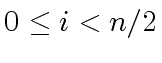. By using Relation (31) with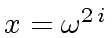we obtain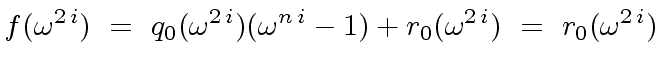(36)

since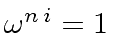. Then, by using Relation (32) with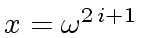we obtain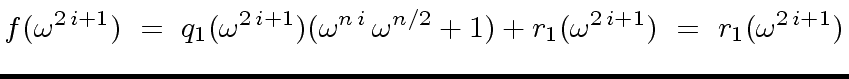(37)

since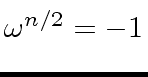. Indeed, this last equation follows from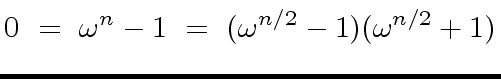(38)

and the fact that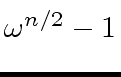is not zero nor a zero divisor. Therefore we have proved the following

Proposition 4   Evaluating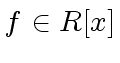(with degree less than) at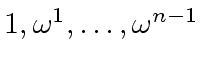is equivalent to
• evaluate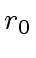at the even powers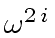for, and
• evaluate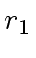at the odd powers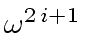for.

Since it is easy to show that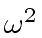is a primitive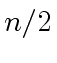-th root of unity we can hope for a recursive algorithm. This algorithm would be easier if bothandwould be evaluated at the same points. So we define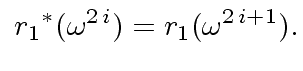(39)

Now we obtain the following algorithm.

Algorithm 1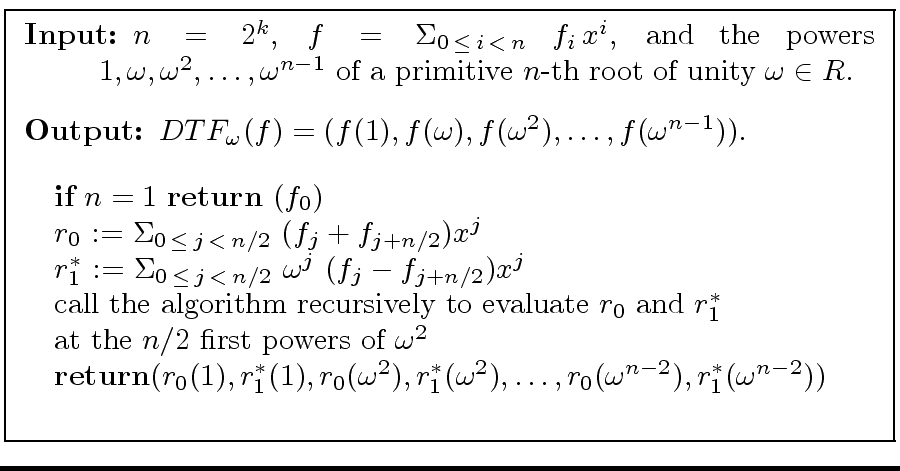Theorem 1   Letbe a power of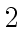andbe a primitive-th root of unity. Then Algorithm 1 computes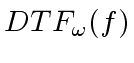using
•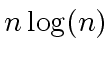additions in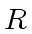,
•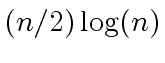multiplications by powers of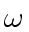.
leading in total to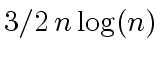ring operations.

Proof. By induction on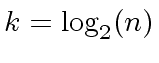. Let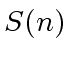and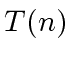be the number of additions and multiplications inthat the algorithms requires for an input of size. If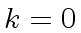the algorithm returns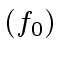whose costs is null thus we have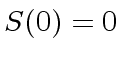and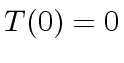which satisfies the formula since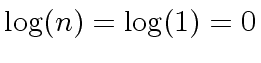. Assume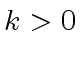. Just by looking at the algorithm we that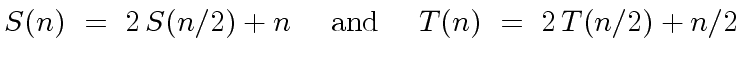(40)

leading to the result by plugging in the induction hypothesis.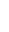Marc Moreno Maza
2008-01-07